4. Method for Estimating the Water/Concrete Ratio Based on the Index of Water Volume

In diagnosis of concrete quality, it is important to understand the water/cement ratio (W/C). However, the method generally used for estimating W/C requires complicated procedures and can be inaccurate. A new method for estimating W/C using geometrical characteristics was therefore proposed.
The size of calcium hydroxide Ca(OH)2 crystals formed in the concrete is in direct proportion to W/C. Thus, W/C can be estimated (Fig. 1) by determining the size of the calcium hydroxide crystals from the ratio (P1 /P2 : Index of water volume) of the diffraction peak of the crystal plane (001) to the crystal plane (101) using powder X-ray diffraction. However, the index of water volume is affected by the quantity and type of aggregate contained in the measuring specimen. Studies were therefore carried out to enable W/C determination in any specimen.
The studies showed that W/C can be determined accurately using a method by which, if the aggregate is not soluble in acid, the index of water volume is measured by the quantity of aggregate remaining after dissolving the specimen in acid. If an aggregate, such as limestone, is soluble in acid, W/C can be determined by finding the quantity of Ca(OH)2 using a thermal analyzer and fitting it to the calibration curve in combination with the index of water volume. By combining the specimen's aggregate quantity or Ca(OH)2 quantity with the index of water volume in this manner, W/C can be estimated more accurately and easily in wider areas.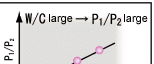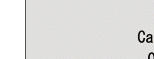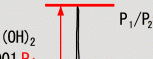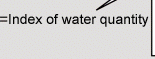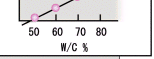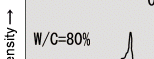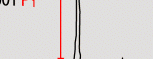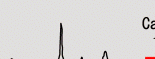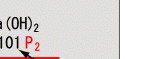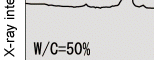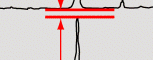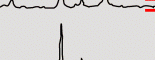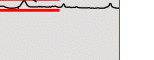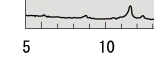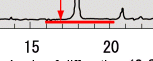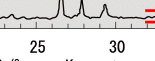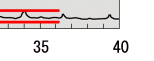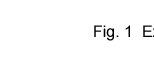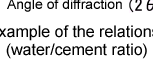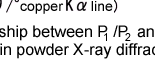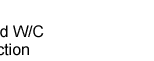HOME > Major Results of Research and Development in Fiscal 2002 > II Cost Effectiveness/Efficiency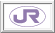RTRI HOME